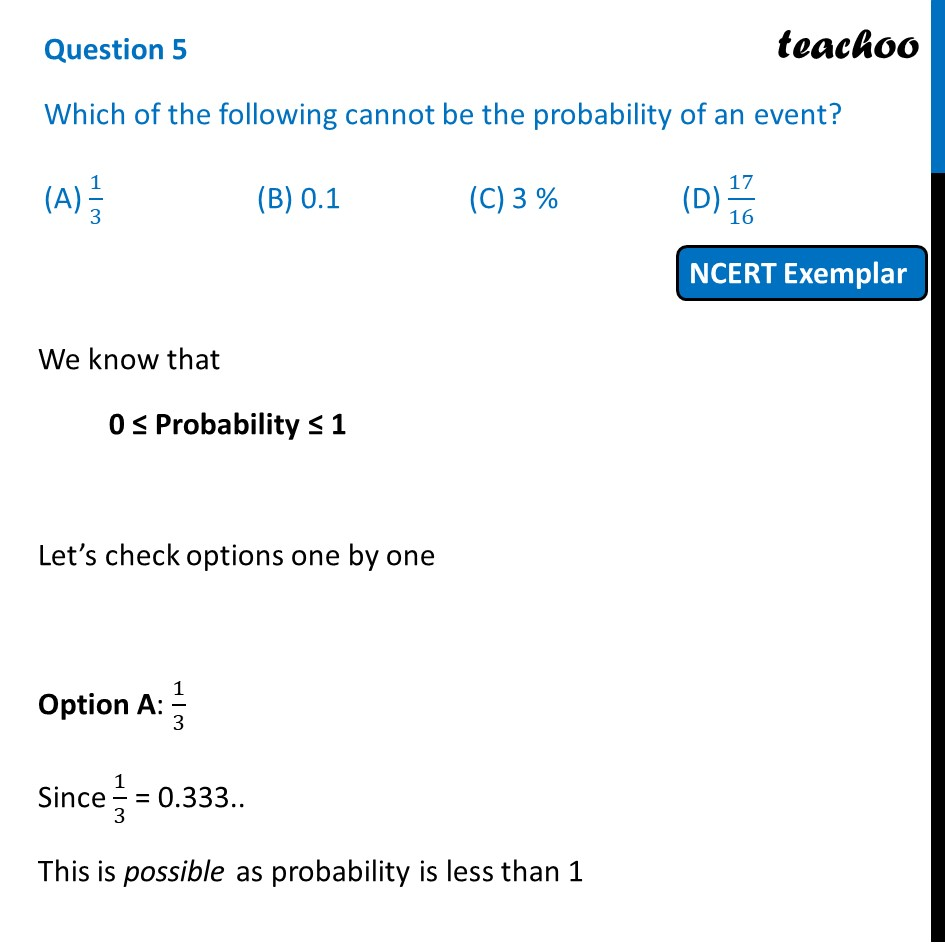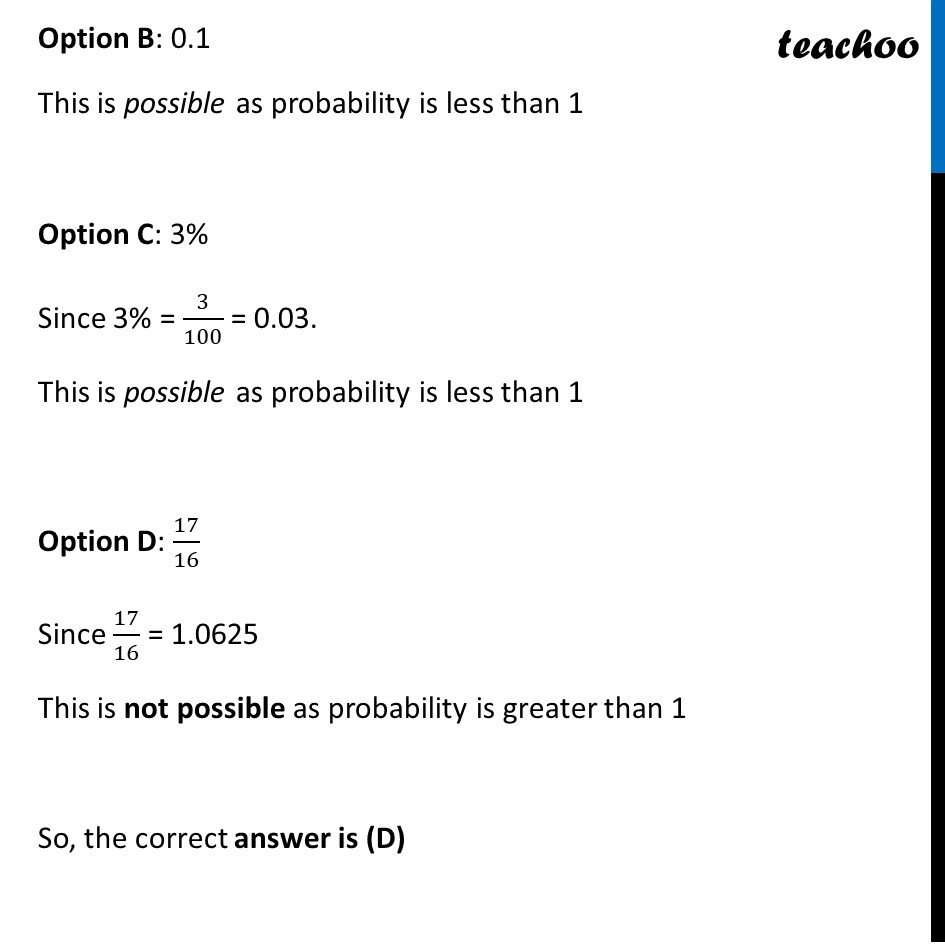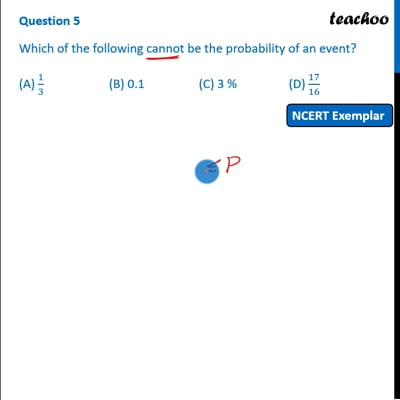NCERT Exemplar - MCQ

Chapter 15 Class 10 Probability
Serial order wise

## (A) 1/3   (B) 0.1  (C) 3 %  (D) 17/16This video is only available for Teachoo black users

Maths Crash Course - Live lectures + all videos + Real time Doubt solving!

### Transcript

Question 5 Which of the following cannot be the probability of an event? (A) 1/3 (B) 0.1 (C) 3 % (D) 17/16 We know that 0 ≤ Probability ≤ 1 Let’s check options one by one Option A: 1/3 Since 1/3 = 0.333.. This is possible as probability is less than 1 Option B: 0.1 This is possible as probability is less than 1 Option C: 3% Since 3% = 3/100 = 0.03. This is possible as probability is less than 1 Option D: 17/16 Since 17/16 = 1.0625 This is not possible as probability is greater than 1 So, the correct answer is (D)